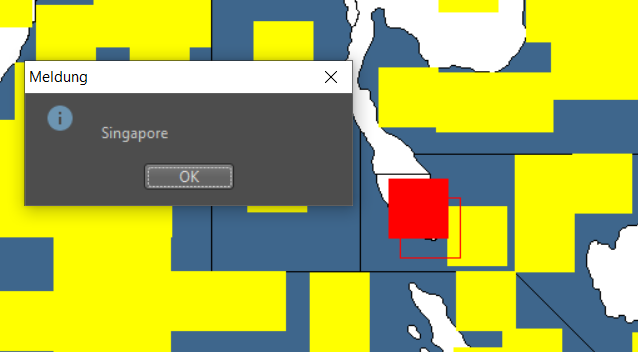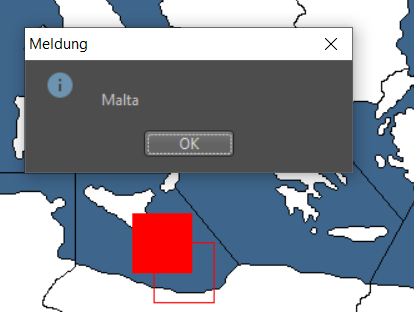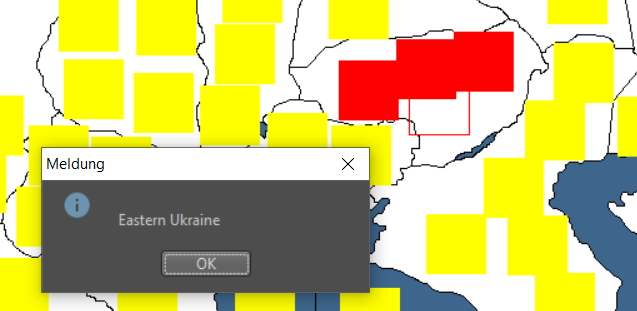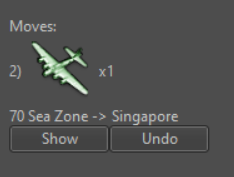# connection problems

<territory name="Singapore"/>
<connection t1="37 Sea Zone" t2="Singapore"/>

but you can't land units from a transporter from 37 to Singapore

1. <connection t1="Caucasus" t2="Eastern Ukraine"/>

but you cant move units from Caucasus to Eastern Ukraine

<territory name="Malta"/>
<territory name="66 Sea Zone" water="true"/>
<connection t1="Malta" t2="66 Sea Zone"/>

but you can't land units from SZ 66 to Malta, neither ait units nor ground units from a transporter

what is the reason for this?

• @Numetalfan Can you attach the entire xml?

• @Numetalfan Idk if it matters but you also have this:

<connection t1="66 Sea Zone" t2="Malta"/>

I checked:
<connection t1="13 Sea Zone" t2="Gibraltar"/>
and it doesn't have a :
<connection t1="Gibraltar" t2="13 Sea Zone"/>

so maybe you only need one or the other.

• @Numetalfan

When you redid the map for your game did you make sure that these new territories had placements set. You may want to rerun the 'Placement Picker' and check.

Cheers...

• from my point of view, all places are fine:• @Numetalfan

With the information provided, there does not seem to be any problems. You have the territories listed, along with the connections. (You only need one set of connections, the other way is not required.) And you have the territory attachment.

The problem may lie in one of the other files, place.txt, centers.txt, etc. Which would require the whole map to find your problem.

Sorry.Cheers...

• centers.txt:

Singapore (2235,1190)
37 Sea Zone (2276,1216)

Eastern Ukraine (1491,425)
Caucasus (1561,520)

Malta (1122,809)
66 Sea Zone (1101,814)

place.txt:

66 Sea Zone (1135,800) (1061,793)
Malta (1105,783)

Eastern Ukraine (1469,402) (1423,419) (1515,396)
Caucasus (1539,497) (1493,542) (1558,543) (1572,451) (1580,589) (1595,405)

Singapore (2216,1170)
37 Sea Zone (2263,1192)

polygons.txt:
37 Sea Zone < (2275,1150) (2316,1150) (2317,1151) (2317,1243) (2316,1244) (2194,1244) (2193,1243) (2193,1133) (2194,1132) (2196,1134) (2196,1135) (2198,1137) (2198,1139) (2201,1142) (2201,1143) (2203,1145) (2203,1146) (2204,1147) (2204,1151) (2203,1152) (2203,1154) (2202,1155) (2202,1157) (2203,1158) (2203,1163) (2204,1164) (2204,1165) (2206,1167) (2206,1173) (2207,1174) (2207,1176) (2208,1177) (2208,1179) (2209,1180) (2209,1181) (2210,1182) (2210,1184) (2211,1185) (2211,1186) (2215,1190) (2215,1192) (2216,1193) (2216,1194) (2218,1196) (2218,1197) (2223,1202) (2224,1202) (2232,1210) (2233,1210) (2236,1213) (2238,1213) (2239,1214) (2239,1215) (2241,1215) (2242,1216) (2243,1216) (2245,1218) (2249,1218) (2250,1219) (2253,1219) (2255,1217) (2255,1216) (2256,1215) (2256,1211) (2255,1210) (2255,1209) (2254,1208) (2254,1206) (2252,1204) (2252,1202) (2251,1201) (2251,1200) (2245,1194) (2245,1184) (2248,1181) (2248,1180) (2249,1179) (2249,1166) (2238,1155) (2238,1154) (2236,1152) (2236,1151) (2237,1150) >
Singapore < (2223,1166) (2248,1166) (2249,1167) (2249,1179) (2248,1180) (2248,1181) (2245,1184) (2245,1194) (2251,1200) (2251,1202) (2252,1203) (2252,1205) (2254,1207) (2254,1209) (2255,1210) (2255,1211) (2256,1212) (2256,1214) (2255,1215) (2255,1216) (2252,1219) (2251,1219) (2250,1218) (2246,1218) (2244,1216) (2243,1216) (2242,1215) (2240,1215) (2238,1213) (2236,1213) (2233,1210) (2232,1210) (2231,1209) (2231,1208) (2230,1208) (2227,1205) (2227,1204) (2226,1204) (2224,1202) (2223,1202) (2220,1199) (2220,1198) (2219,1198) (2218,1197) (2218,1196) (2216,1194) (2216,1193) (2215,1192) (2215,1190) (2211,1186) (2211,1185) (2210,1184) (2210,1182) (2209,1181) (2209,1180) (2208,1179) (2208,1177) (2207,1176) (2207,1174) (2206,1173) (2206,1167) (2207,1166) >

Eastern Ukraine < (1488,399) (1489,399) (1490,398) (1492,398) (1495,395) (1506,395) (1507,394) (1513,394) (1515,396) (1539,396) (1541,394) (1542,394) (1543,393) (1544,393) (1545,392) (1546,392) (1547,391) (1549,391) (1550,390) (1551,390) (1552,389) (1554,389) (1558,385) (1559,386) (1562,386) (1563,387) (1565,387) (1566,388) (1566,389) (1567,390) (1567,392) (1568,392) (1569,393) (1570,393) (1576,399) (1576,400) (1581,405) (1581,406) (1583,408) (1583,409) (1584,410) (1584,412) (1585,413) (1585,416) (1586,417) (1586,418) (1587,419) (1587,420) (1588,421) (1589,421) (1590,422) (1590,425) (1589,426) (1588,426) (1585,429) (1584,428) (1584,426) (1579,426) (1577,428) (1577,429) (1576,430) (1576,433) (1574,435) (1574,445) (1573,446) (1573,449) (1571,451) (1571,453) (1570,454) (1570,458) (1569,459) (1569,460) (1567,462) (1566,462) (1565,463) (1565,467) (1564,468) (1563,468) (1562,469) (1561,469) (1558,472) (1554,472) (1550,476) (1547,476) (1546,477) (1545,477) (1543,479) (1542,479) (1537,484) (1537,485) (1535,487) (1533,487) (1532,488) (1531,488) (1530,489) (1529,489) (1529,491) (1528,492) (1523,492) (1522,493) (1520,493) (1519,494) (1518,494) (1516,496) (1516,497) (1514,499) (1513,499) (1512,500) (1511,500) (1510,501) (1509,501) (1506,504) (1502,504) (1501,503) (1496,503) (1495,502) (1489,502) (1488,503) (1482,503) (1481,502) (1481,499) (1483,497) (1483,494) (1481,492) (1481,490) (1482,489) (1487,489) (1487,479) (1486,479) (1484,477) (1484,475) (1483,474) (1483,469) (1481,467) (1479,467) (1478,466) (1477,466) (1476,465) (1469,465) (1468,466) (1467,466) (1467,467) (1466,468) (1462,468) (1461,467) (1461,466) (1459,466) (1457,468) (1456,468) (1455,469) (1454,469) (1454,470) (1453,471) (1450,471) (1449,470) (1444,470) (1442,472) (1439,472) (1438,471) (1435,471) (1434,470) (1431,470) (1430,469) (1427,469) (1426,468) (1422,468) (1421,467) (1418,467) (1417,466) (1414,466) (1413,465) (1412,465) (1411,464) (1411,463) (1410,462) (1410,459) (1409,458) (1409,457) (1408,456) (1408,454) (1405,451) (1395,451) (1394,450) (1392,450) (1391,449) (1391,446) (1392,445) (1392,442) (1391,441) (1391,439) (1390,439) (1389,438) (1389,437) (1388,436) (1388,435) (1387,434) (1387,433) (1386,432) (1386,431) (1385,430) (1384,430) (1383,429) (1383,425) (1384,424) (1397,424) (1404,417) (1404,416) (1412,408) (1418,408) (1419,407) (1420,407) (1421,406) (1422,406) (1423,405) (1424,405) (1426,403) (1428,403) (1429,402) (1430,402) (1431,401) (1433,401) (1434,400) (1442,400) (1443,401) (1468,401) (1469,400) (1481,400) (1482,399) >
Caucasus < (1525,496) (1530,496) (1534,492) (1535,492) (1539,488) (1541,488) (1541,487) (1542,486) (1543,486) (1543,485) (1544,484) (1545,484) (1547,482) (1547,480) (1548,479) (1548,478) (1550,476) (1551,476) (1553,474) (1553,473) (1554,472) (1559,472) (1562,469) (1563,469) (1564,468) (1565,468) (1565,464) (1567,462) (1568,462) (1569,461) (1569,460) (1570,459) (1570,454) (1571,453) (1571,452) (1572,451) (1572,450) (1573,449) (1573,446) (1574,445) (1574,435) (1575,434) (1576,434) (1576,431) (1577,430) (1577,429) (1580,426) (1583,426) (1584,427) (1584,429) (1585,429) (1586,428) (1587,428) (1590,425) (1590,421) (1589,421) (1587,419) (1587,418) (1586,417) (1586,416) (1585,415) (1585,413) (1586,412) (1588,412) (1588,410) (1590,408) (1591,408) (1593,406) (1594,406) (1596,404) (1606,404) (1608,402) (1609,402) (1611,400) (1612,400) (1614,398) (1628,398) (1629,399) (1635,399) (1636,400) (1638,400) (1642,404) (1642,406) (1643,407) (1643,408) (1644,409) (1657,409) (1658,410) (1658,412) (1659,413) (1660,413) (1661,414) (1662,414) (1666,418) (1666,419) (1667,420) (1667,421) (1665,423) (1665,424) (1660,429) (1660,430) (1655,435) (1655,436) (1650,441) (1650,442) (1645,447) (1645,448) (1642,451) (1642,456) (1641,457) (1641,466) (1640,467) (1640,476) (1639,477) (1639,486) (1638,487) (1638,492) (1637,493) (1637,494) (1635,496) (1635,497) (1634,498) (1633,498) (1632,499) (1619,499) (1617,501) (1615,501) (1614,500) (1607,500) (1605,502) (1604,502) (1604,505) (1601,508) (1599,508) (1598,509) (1598,515) (1596,517) (1595,517) (1594,518) (1594,523) (1595,524) (1595,528) (1594,529) (1593,529) (1592,530) (1591,530) (1591,534) (1594,537) (1597,537) (1598,538) (1599,538) (1600,539) (1601,539) (1603,541) (1603,542) (1605,544) (1606,544) (1607,545) (1607,548) (1609,550) (1609,553) (1611,555) (1611,559) (1612,559) (1614,561) (1614,562) (1615,563) (1615,564) (1616,564) (1617,565) (1621,565) (1623,567) (1623,568) (1624,569) (1624,570) (1626,572) (1626,573) (1627,574) (1627,576) (1637,586) (1638,586) (1643,591) (1644,591) (1645,592) (1646,592) (1649,595) (1649,598) (1647,600) (1647,601) (1646,602) (1646,603) (1645,604) (1645,617) (1644,618) (1644,622) (1642,624) (1642,632) (1643,633) (1643,634) (1645,636) (1645,640) (1646,641) (1646,642) (1648,644) (1647,645) (1639,645) (1635,641) (1635,636) (1634,635) (1633,635) (1632,634) (1631,634) (1630,633) (1629,633) (1628,634) (1627,634) (1626,635) (1625,635) (1624,636) (1624,637) (1623,638) (1623,640) (1621,642) (1603,642) (1601,644) (1589,644) (1588,643) (1587,643) (1586,642) (1586,641) (1585,641) (1584,640) (1583,640) (1582,639) (1573,639) (1572,638) (1571,638) (1569,636) (1566,636) (1565,635) (1561,635) (1559,633) (1542,633) (1536,627) (1534,627) (1533,626) (1534,625) (1534,620) (1533,619) (1533,618) (1522,607) (1521,607) (1520,606) (1518,606) (1517,605) (1515,605) (1514,604) (1503,604) (1502,603) (1500,603) (1499,602) (1498,602) (1493,597) (1490,597) (1489,596) (1488,596) (1486,594) (1485,594) (1484,593) (1483,593) (1482,592) (1480,592) (1479,591) (1478,591) (1477,590) (1476,590) (1474,588) (1473,588) (1472,587) (1470,587) (1469,586) (1467,586) (1466,585) (1457,585) (1456,584) (1455,584) (1452,581) (1446,581) (1443,578) (1443,574) (1444,573) (1449,573) (1451,571) (1451,565) (1452,564) (1452,563) (1453,562) (1455,562) (1455,556) (1456,555) (1458,555) (1459,554) (1460,554) (1460,551) (1455,551) (1453,549) (1451,549) (1450,548) (1450,545) (1454,541) (1457,541) (1458,540) (1458,539) (1459,538) (1459,536) (1462,533) (1463,533) (1463,530) (1462,529) (1457,529) (1454,532) (1452,532) (1451,531) (1451,529) (1447,525) (1447,522) (1450,519) (1451,519) (1451,518) (1452,517) (1458,517) (1459,518) (1469,518) (1469,515) (1470,514) (1473,514) (1474,513) (1474,509) (1472,507) (1472,506) (1473,505) (1477,505) (1478,504) (1479,504) (1480,503) (1488,503) (1489,502) (1495,502) (1496,503) (1501,503) (1502,504) (1507,504) (1507,503) (1508,502) (1509,502) (1511,500) (1512,500) (1513,499) (1515,499) (1516,498) (1521,498) (1522,497) (1523,497) (1524,496) >

Malta < (1123,812) (1127,812) (1127,811) (1128,810) (1128,806) (1127,805) (1121,805) (1120,806) (1120,807) (1119,808) (1118,808) (1118,810) (1119,810) (1121,812) >
66 Sea Zone < (1158,785) (1158,785) (1161,788) (1161,789) (1165,793) (1165,794) (1170,799) (1170,800) (1175,805) (1175,806) (1179,810) (1179,811) (1184,816) (1184,817) (1189,822) (1189,824) (1180,833) (1180,834) (1179,835) (1179,838) (1174,843) (1169,843) (1168,844) (1164,844) (1162,846) (1140,846) (1139,845) (1138,845) (1137,844) (1136,844) (1135,843) (1130,843) (1129,842) (1120,842) (1119,841) (1118,841) (1117,840) (1116,840) (1115,839) (1114,839) (1113,838) (1108,838) (1107,837) (1106,837) (1105,836) (1104,836) (1102,834) (1100,834) (1099,833) (1098,833) (1097,832) (1095,832) (1094,831) (1085,831) (1084,830) (1080,830) (1079,829) (1077,829) (1076,828) (1075,828) (1074,827) (1072,827) (1070,825) (1068,825) (1067,824) (1062,824) (1061,823) (1060,823) (1059,822) (1059,751) (1060,750) (1133,750) (1134,751) (1133,752) (1133,753) (1130,756) (1130,758) (1129,759) (1128,759) (1127,760) (1127,763) (1128,764) (1129,764) (1131,766) (1132,766) (1134,764) (1137,764) (1139,762) (1142,765) (1142,766) (1147,771) (1147,772) (1152,777) (1152,778) (1156,782) (1156,783) >

connections.txt:

<connection t1="66 Sea Zone" t2="Malta"/>
<connection t1="Caucasus" t2="Eastern Ukraine"/>
<connection t1="37 Sea Zone" t2="Singapore"/>

--

any other file to check?

• I would try eliminating the doubled connections like

``````    <connection t1="Caucasus" t2="Eastern Ukraine"/>
<connection t1="Eastern Ukraine" t2="Caucasus"/>

``````

It is not necessary and may be confusing the program in some fashion.

The scenario looks interesting. I always like playing on the smaller maps.

• found a bug - but not the reason for itthis unit move should be impossible, as there is no connection between SZ 70 and Singapore is definied in the xml.

• @Numetalfan Try cleaning up all the doubled connections. That may be causing all the problems.

• Hi,

all problems are gone.

The trick - I started a new game and everything worked well.
However - our saved game is still c´broken, there all connection problems are still alive.

Strange.

• @RogerCooper

first, thx for the hints. I cleaned up my xml removed all the double entries for connections

secondly, thx for the compliment. We are still busy working on that szenario, it is not yet finished. The idea of a sploit of the US is a nice thing, it makes the game much more interesting as there is always action in both the Atlantic and the Pacific,
however there is al lot to do too. new national objectives, balancing the map and the units, the starting income etc.

42388
2057
2291
Who's Online
Visitors Today